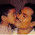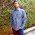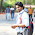## January 28, 2015

### Puzzle: Wire Delay

Technical Tidbit:

You have a long wire of let's say length L. And it has the net delay of 100ps. Now you split the wire into two equal halves of length 0.5L each and insert a buffer at the center of the wire. The delay of buffer is 25ps. What is the total delay of the system: (half wire+ buffer + half wire)?

Option a: 50+25+50= 125ps
Option b: 25+25+25=75ps
Option c: 25+25+50=100ps
Option d: 50+25+25=100ps

Choose the right option, and please give a short explanation.

Thanks!

#### 10 comments:

1.Option b. Currently studying this so I wish to try it out. I remember wire delay's proportional to the square of the length (0.38RCL^2) so if we split the wire into half the delay goes down from 100ps for L to 25ps for 0.5L. Then the total delay is just the sum of the buffer plus two 0.5L wires.

1.This comment has been removed by the author.

2.Yes, I do agree with Chirag’s comment

If R0 is the unit resistance and C0 is the unit capacitance
Total delay of wire of unit length = R0 L x C0 L
= R0C0 L^2

As this buffer dividing the net into equal half’s i.e. L/2

Now delay offered by wire = R0C0 (L/2)^2 + R0C0 (L/2)^2
= R0C0 (L^2/4) + R0C0 (L^2/4)
= 25 + 25

Total Delay of system = 25 + 25 + 25

2.This comment has been removed by the author.

1.Over all system delay, depends on kind of buffer you add too, in this case a 100ps buffer will increase the overall delay.

2.Also, you cannot divide R and C, its the length that should get divided.

3.Lets take this,
T=R.C
R= kL/A
so T directly proportional to R & R is directly proportional to L
so T is directly proportional to L
so 50+25+50=125ps

Give me explanation if I am wrong..!

1.Your approach is right! But you are missing C component change in your equation. If you consider that too, answer would come out as option b.

4.yes R prop to L..den c prop to L..so T prop to L^2..

5.so option b. .